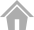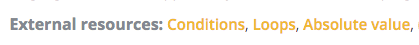# Temperatures puzzle discussion

#694

(PHP) Tous les tests passent dans la page de code à 100%. et seulement 85 % après soumission sur question 2 et 3.
(PHP) all code example passed in dev hud. but after submiting only 85 % . any clue ?

#695
`````` int CT=abs(temp);
int j=sizeof(temp);
for(int i=0;i<n;i++)
{
if(CT>abs(temp[i]))
{
CT=abs(temp[i]);
p=i;

}
}
for(int i=0;i<n;i++)
{
if(CT==abs(temp[i]))
{
if(temp[p]<temp[i])
{
CT=temp[i];
}
}
else
{
CT=temp[p];
}
}
``````

whats the problem with this code i cant get through the the 3 test case everything else does work

#696

I advise you to add “error prints” to your algorithm to know the values of CT and p for example. You’ll quickly see the mistake in your code. Good luck!

#697

Thanks! That did it. My code has a section that tests if the input is numeric, and sets the output to zero if that’s the case. The initial input values are string values, not integers. I ran intval() on the input value first, and it works now.

#698

I am stuck… I don’t know where is the bug.Can anyone help me out?

/**
* Auto-generated code below aims at helping you parse
* the standard input according to the problem statement.
**/

``````const n = parseInt(readline()); // the number of temperatures to analyse
var inputs = readline().split(' ');
var arr=[];
for (let i = 0; i < n; i++) {
var t = parseInt(inputs[i]); // a temperature expressed as an integer ranging from -273 to 5526
if(t<0){return 1}
arr.push(t);

}

var closeToZero=Math.min(...arr);
// if(closeToZero<0){return 1}

// Write an action using print()
// To debug: printErr('Debug messages...');

print('closeToZero');``````

#699

You have to print an integer value, not a string.

#700

I dont understant the input of this programm.

We have n = nmbrs of temperature : n = int(raw_input()) ok
And we must have a string with the n temperatures, where is it ?
do we have to write it ?

Also I dont understant that line
for i in raw_input().split():
# t: a temperature expressed as an integer ranging from -273 to 5526
t = int(i)

because in this case t will be 1, 2 ,3, 4 , …

Why is it to be a string ? will not be better a list ?
So after we can take the min, of abs of the values in this list.

Thanks for your help

#701

Hey there I need some help in this,

I will revisit this later in the day that i am writing this but some tips or subtle hints would be greatly appreciated. This is logic that i have in the standard loop for the problem.

``````        if(i >= 1){
if(Math.abs(pastNum) < Math.abs(t)){
result = String.valueOf(pastNum);
}

else if (Math.abs(t) < Math.abs(pastNum)) {
result = String.valueOf(t);
}

else if (Math.abs(pastNum) == Math.abs(t)){
if(pastNum > 0){
result = String.valueOf(pastNum);
}
else if (t > 0){
result = String.valueOf(t);
}

else{
result = String.valueOf(t);
}
}
}

else{
pastNum = t;
}
``````

Thanks again.

#702

So guys, I’m a total noob and trying to solve this… I’ve managed to get through most of the tests with the code I wrote (though it’s probably not the most convenient way) But I don’t see how I can pick the positive temperature instead of the negative one, any help on this?

my code:

``````#include <iostream>
#include <string>
#include <vector>
#include <algorithm>

using namespace std;

/**
* Auto-generated code below aims at helping you parse
* the standard input according to the problem statement.
**/
int main()
{
int zero = 0;
int result = 0 ;
int n; // the number of temperatures to analyse

cin >> n; cin.ignore();

//loop temperatures on row
for (int i = 0; i < n; i++) {
int t; // a temperature expressed as an integer ranging from -273 to 5526
cin >> t; cin.ignore();

if(i == 0 || (t < zero && ((zero - result) > (zero - t))) || ((t > zero && (zero + result) > (zero + t)))) {
result = t;}

}

// Write an action using cout. DON'T FORGET THE "<< endl"
// To debug: cerr << "Debug messages..." << endl;

cout << result << endl;``````

#703#704

Many thanks, did not know about the sqrt() function. Had to include the <math.h> library though. I’ve changed my if statement to the following and now it’s working!

``````if(sqrt(t*t) == sqrt(result*result)){
result = sqrt(result*result);
}
else if(i == 0 || (t < zero && ((zero - result) > (zero - t))) || ((t > zero && (zero + result) > (zero + t)))) {
result = t;}``````

#705

If you solved it to 100%, you have now access to published solutions (https://www.codingame.com/training/easy/temperatures/solution). I’m sure you will find more tricks to learn.

#706

Thanks, did not know about that yet. I now managed to get the abs() function in, instead of calculating the absolute integer myself. However, many of the functions I see in the solutions I haven’t familiarized myself yet, so I guess I’ll have to learn a little more before I can fully understand them, thanks again!

#707

Hi, I am using python3.

I haven’t been able to do any of the test case with any of the codes I have written. It always returns the furthest number. This is what I have.

``````import sys
import math

# Auto-generated code below aims at helping you parse
# the standard input according to the problem statement.

n = int(input())  # the number of temperatures to analyse

# print(type(input())) # String

if n == 0:
closest=0
else:
for i in (input().split()):
# t: a temperature expressed as an integer ranging from -273 to 5526
t = int(i)
closest = 5527
if abs(t)<int(closest):
closest = t

# Write an action using print
# print("Debug messages...", file=sys.stderr)

print(closest)``````

#708

You can initialize closest with float(“inf”).
Moreover, “-5 5” will fail but “5 -5” will succeed.

#709

My code works well on any other website, but it’s not working on CodinGame. It works for 1,2,4, but it tells me it returns “12” for #3 and “-4” for #5. Any thoughts?

My code:

``````    n = int(input())  # the number of temperatures to analyse

templist = {}
realtemp = []
abstemp = []
newlist =[]

for i in input().split():
# t: a temperature expressed as an integer ranging from -273 to 5526
a = int(i) # log the
t = (abs(int(i)))
realtemp.append(a)
abstemp.append(t)

def dedupe(list):
for m in list:
if (list.count(m)+list.count(-m))>1:
list[m]=abs(m)
return list

newtemp = dedupe(realtemp)

templist= dict(zip(newtemp,abstemp))
answer = min(templist, key=templist.get)``````

#710

it seems you just need to implement the following rule to validate all test cases:

If two numbers are equally close to zero, positive integer has to be considered closest to zero (for instance, if the temperatures are -5 and 5, then display 5).

#712

does any one know why (b) keep sending 0 as output ?
where and how to declare b to prevent this from happening?

``````#include <stdlib.h>
#include <stdio.h>
#include <string.h>

/**
* Auto-generated code below aims at helping you parse
* the standard input according to the problem statement.
**/
int main()
{
int n; // the number of temperatures to analyse
scanf("%d", &n);
for (int i = 0; i < n; i++) {
int t;
// a temperature expressed as an integer ranging from -273 to 5526
scanf("%d",&t);
printf("t=%d\n",t);// just for debugging
if (abs(t)<b){b=t;}
printf("b=%d\n",b);
if (n==i) {printf("%d\n",b);}
}

// Write an action using printf(). DON'T FORGET THE TRAILING \n
// To debug: fprintf(stderr, "Debug messages...\n");

//printf("%d\n",b);

return 0;
}
``````

the output

``````### Standard Output Stream:

t=1

b=0

t=-2

b=0

t=-8

b=0

t=4

b=0

t=5

b=0

### Failure

Found:

t=...

Expected:

1``````

#714

B equals 0, everything is greater than it. you should initialise b to 9999 at the same place you initialise n.